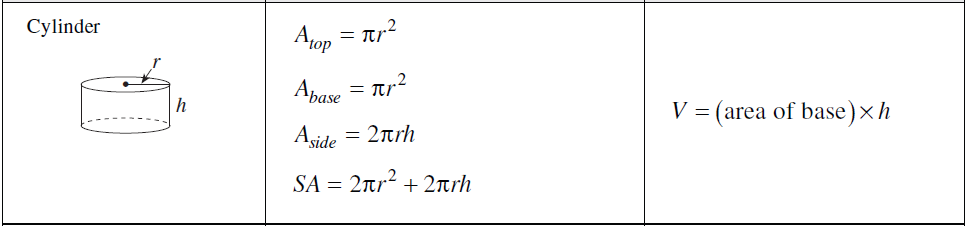# Surface area and volume of cylinders#### Everything You Need in One Place

Homework problems? Exam preparation? Trying to grasp a concept or just brushing up the basics? Our extensive help & practice library have got you covered.#### Learn and Practice With Ease

Our proven video lessons ease you through problems quickly, and you get tonnes of friendly practice on questions that trip students up on tests and finals.#### Instant and Unlimited Help

Our personalized learning platform enables you to instantly find the exact walkthrough to your specific type of question. Activate unlimited help now!

0/0
0/2
##### Examples
###### Lessons
1. Find the surface area and the volume of the following cylinders:

1.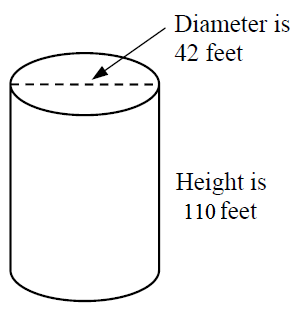2.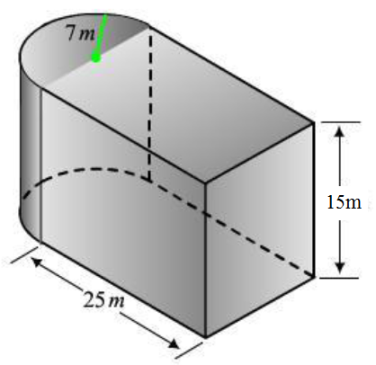###### Free to Join!
StudyPug is a learning help platform covering math and science from grade 4 all the way to second year university. Our video tutorials, unlimited practice problems, and step-by-step explanations provide you or your child with all the help you need to master concepts. On top of that, it's fun - with achievements, customizable avatars, and awards to keep you motivated.
• #### Easily See Your ProgressWe track the progress you've made on a topic so you know what you've done. From the course view you can easily see what topics have what and the progress you've made on them. Fill the rings to completely master that section or mouse over the icon to see more details.
• #### Make Use of Our Learning Aids###### Practice Accuracy

Get quick access to the topic you're currently learning.

See how well your practice sessions are going over time.

Stay on track with our daily recommendations.

• #### Earn Achievements as You LearnMake the most of your time as you use StudyPug to help you achieve your goals. Earn fun little badges the more you watch, practice, and use our service.
• #### Create and Customize Your AvatarPlay with our fun little avatar builder to create and customize your own avatar on StudyPug. Choose your face, eye colour, hair colour and style, and background. Unlock more options the more you use StudyPug.
###### Topic Notes
A cylinder is like stacking up a pile of circle plates. With information such as, height, radius, and circumference, it is easy to calculate the surface area and volume of cylinders.Let's look at how to calculate the surface area and volume of composite solids too.

## Surface area and volume of a cylinder using simple formulas

Cylinders are shaped like a tube, with two circles at both ends that are identical and parallel. In geometry, you'll be required to use formulas in order to find the volume and surface area of this shape. You will encounter problems that may not provide you with all the info you'll need to easily plug in numbers into the formulas, and that's where your previous knowledge of geometry will come into play.

## How to find the surface area of a cylinder

We'll teach you how to find the surface area and the volume of cylinders through an example question. This is not too difficult as we have a nice equation for finding both of these. So for the cylinder in the top left corner below, let's find the surface area first.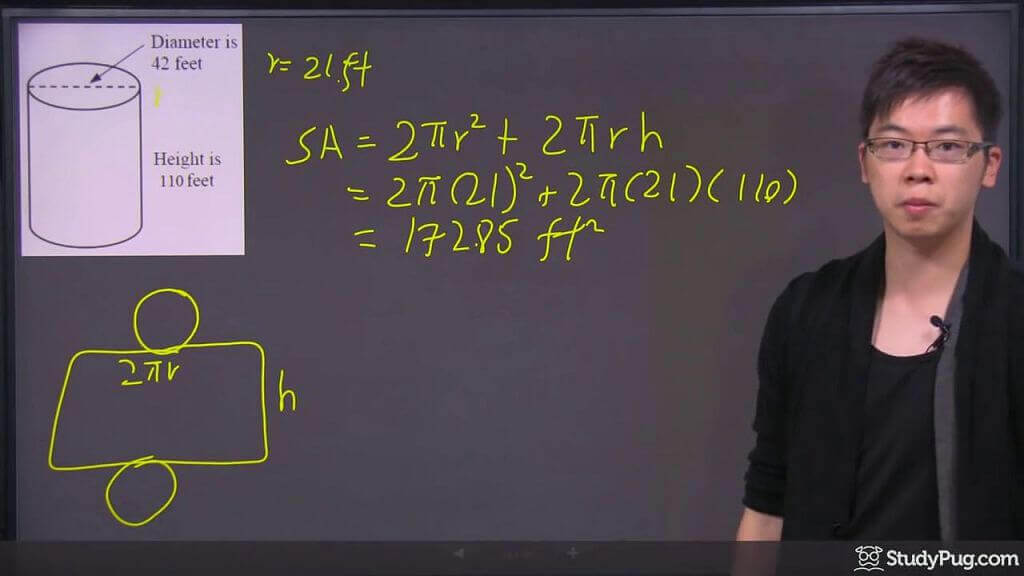steps of finding surface area of a cylinder

To start off, we know that the diameter is equaled to 42 feet and the height is 110 feet. The units are consistent with one another, which is good. But we don't actually need the diameter all that much when it comes to the equation. Instead, we're going to find the radius using the diameter.

If you recall, it's simple to convert between a radius and diameter. In this case, just take the 42 and divide it by 2 and you get the radius which is equaled to 21 feet. Now we can take this and use it in the surface area formula.

## Surface area of a cylinder formula

To begin calculating the surface area of a cylinder, we know that the equation says to take $2 \pi r$-squared, then add $2 \pi r$ with the $h$, which is height.

What is the idea behind this formula? It's actually pretty straightforward. If you were to unwrap a cylinder, you will see that it's a circle on the top with a rectangular shape in the middle and followed by another circle. We've got a total of two circles and one rectangle. A circle's area is equal to $\pi r$ squared, and thus, with two circles, it is $2 \pi r$ squared. The $2 \pi r$ from the second part of the surface area equation is to find the circumference of the circle, which also happens to be the length of the rectangle. It'll help us find the surface area of the rectangle, which is the height multiplied by length, and therefore equal to $2 \pi r h$.

Now that we understand where the equation comes from, let's just plug the numbers that we do know into the equation. What is the cylinder surface area?

You should get:

Surface area

$= 2\pi r^2 + 2\pi rh$

= $2 \pi (21)^2+2 \pi (21)(110)$

=$17285 feet^2$

We've got the radius from the diameter previously (21 feet), and then we just use the height that was given to us, which was 110.

## Volume of a cylinder formula

Before we begin finding the volume, the below shows the volume equation that we'll be using. The volume of a cylinder is simply the area of the base multiplied with the height.

## How to find the volume of a cylinder

Going back to the diagram of the cylinder, the base is circular. The top piece is also circular and identical to the base. We know that the area of a circle is going to be $\pi r$-squared. The height is given, and we know it's once again, 110 feet. What is the cylinder volume?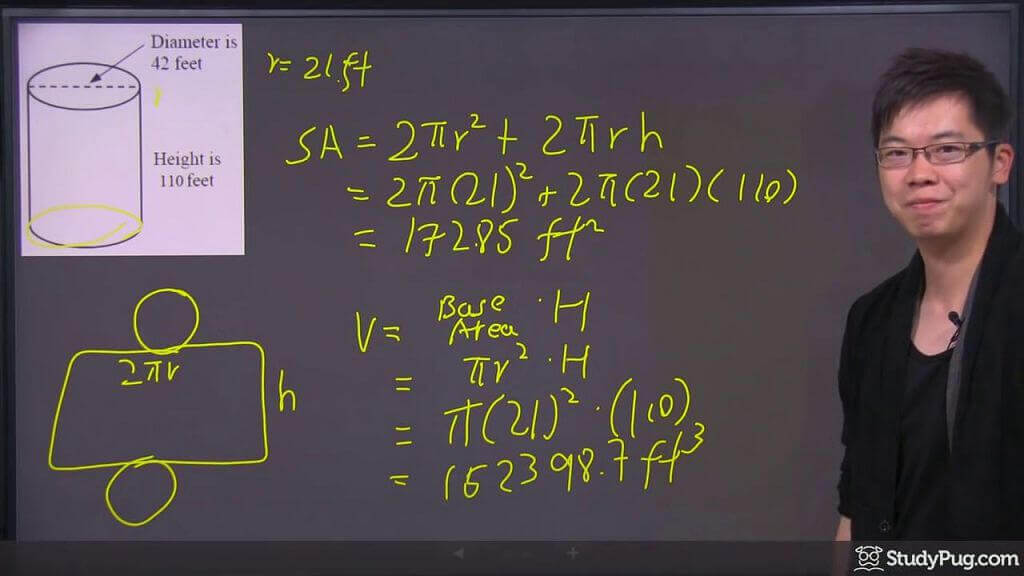steps of finding volume of a cylinder

Let's quickly fill in the information that we have and you should get $152398.7 feet^3$. Remember that volume is in cubes, and that the surface area is in squares. Now you've learned how to calculate the surface area and volume of a cylinder!

To see an interactive example of a cylinder's volume, try out this adjustable figure.